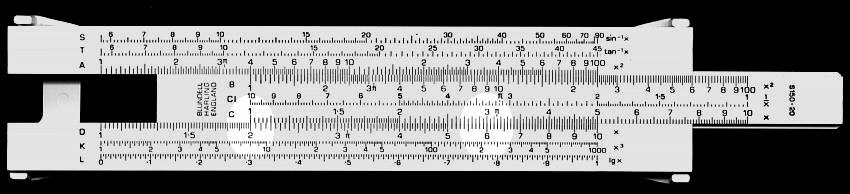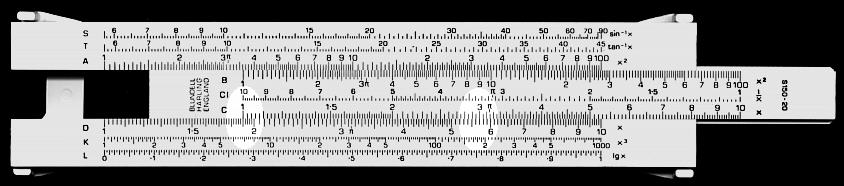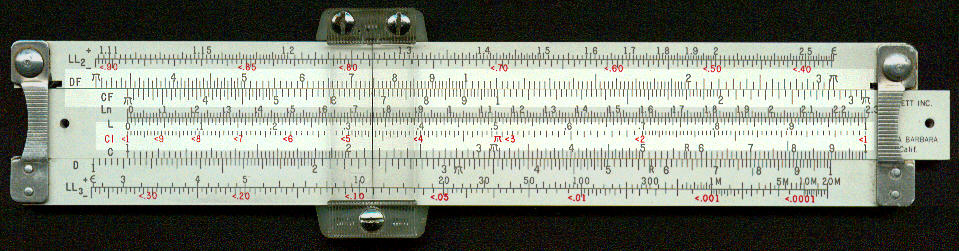Multiplication and division

In this, and descriptions of the use of other scales we only concern ourselves with use of the scales. It is no part of the function of this site to introduce the reader to arithmetic, algebra or trigonometry. We also give a limited number of examples of each type of operation. In the past, text books would give several examples. Since, however, most users of a slide rule will have access to an electronic calculator it will be easy for the reader to devise other examples

All multiplication and division operations can be performed with the C and D scales and these will be described first. Some operations can be performed with less movement of the slide and/or cursor using CI, CF, DF and other scales. These will be described later.

Simple examples

To multiply 2 times 3.

• Position the 1 of the C scale (on slide) against the 2 on the D scale (on stock).
• Move the cursor to 3 on the C scale.To divide 5.7 by 3.

• Move the cursor to 5.7 on the D scale
• Position the 3 on the C scale against the 5.7 on the D scale.
• Read the answer (1.9) on the D scale against the 1 on the C scale.In these examples the cursor has been removed to improve clarity.

More complex example

Let us now consider 3 times 7. If we start as above, we would place the 1 of the C scale against the 3 of the D scale but then we find we cannot move the cursor to the 7 on the C scale as it off the end of the stock. To get around this:

• Place the 10 of the C scale against the 3 on the D scale.
• Move the cursor to the 7 on the C scale.

This appears to be the same answer as in the previous example and shows two things:
1. It is sometimes necessary to use the 10 on the C scale, rather than the 1, to ensure that the results are not off the end of the stock.
2. The position of the decimal point is not always obvious.

As we have seen above, 2.1 in this case represents 21 but, in other calculations, it could equally represent 210 or 0.21. The easiest way around this is to repeat the calculation using approximate values. For example to divide 2100 by 52.

• Position the cursor on 2.1 on the D scale.
• Position the 5.2 on the C scale against the 2.1 on the D scale.
• Read the answer (4.04) on the D scale against the 10 on the C scale.
• Perform a simple approximate calculation, say 2000 / 50. The answer to this is 40.
• This shows that the answer to this calculation is therefore 40.4, not 4.04.

There are rigorous methods for working out the position of the decimal point and some slide rules have symbols to remind users of these methods. Whilst a summary of the methods is given in this site, the method suggested above, a quick approximate calculation, is the fastest and most reliable.

Combined multiplication and division

When dealing with combined multiplication and division the usual order is division, multiplication, division, multiplication...

For example, let us consider  3 * 4 / 1.5. The order would be 3 divided by 1.5 times 4.

• Cursor to 3 on D scale.
• 1.5 on C scale to cursor (=3 on D)
• Cursor to 4 on C scale.
• Answer (8) under cursor on D scale.

It was not necessary to note the values of intermediate stages of the calculations. For example after dividing 3 by 1.5 the result, 2, was not noted.

Let us now consider another example, 3 * 7 / 1.5.

• Cursor to 3 on D scale.
• 1.5 on C scale to cursor (= 3 on D).
• Cursor to 2 on D scale.
• 10 on C scale to cursor (= 2 on D).
• Cursor to 7 on C scale

In this example we have had to move the slide from one end to the other, as we did in one of the multiplication examples above.

Multiplication by a constant - C and D scales

A typical example of this is conversion of units, for example to convert from inches to centimetres (1 inch is 2.54 centimetres).

• Cursor to 2.54 on D scale.
• 1 on C scale to cursor.
• Read centimetres on the D scale against the inches on the C scale. (e.g 2 inches equals 5.08 cm, 3.5 inches equals 8.89 cm).
• For values of inches greater than 4 move the 10 of the C scale to 2.54 and read the answers on the D scale. (e.g. 6 inches equals 15.2 cm)

Use of CI scale

The numbers on the CI scale are the inverse or the reciprocal of those on the C scale. If you have a look at rule with a CI scale you will see the numbers run in the opposite direction to the C scale; they are in effect a mirror image of the C scale. The reciprocal of a number is one divided by the number itself. The reciprocal of 2 for example is 1/2, in other words 0.5. If you move the cursor to 2 on the C scale you will see it lines up with 0.5 (read as 5) on the CI scale.

Whilst the scale can be used for direct calculation of reciprocals, its main advantage is that it can make some calculations easier.

The rule below shows a CI scale with the numbers marked in red.If we want to calculate a/b we can also calculate it as a * (1/b); that is the division of two numbers is the equivalent of the first number multiplied by its reciprocal. Similarly a * b can be written as a / (1/b);  that is the multiplication of two numbers is the equivalent of the first number divided by the reciprocal of the second number.

An example used above was to multiply 3 times 7. When we did that we had to move the slide from end to another. Let us now look at the same calculation using the CI scale.

• Cursor to 3 on D scale
• 7 on the CI scale to the cursor.
• Read the answer 21 against the 1 on the D scale.

You will note that we have used the equivalent movements for a division to perform a multiplication. We have also been able to avoid the need to move the slide from one end to another.

Let us now look at the same example used above, 3 * 7 / 1.5, but using the CI scale.

• Cursor to 3 on D scale.
• 1.5 on C scale to cursor (=3 on D) [These movements are the same as above.]
• Cursor to 1 on the C scale.
• 7 on CI scale to cursor.

Again we have been able to avoid the need to move the cursor from one end to another.

Use of CF and DF scales

These scales are so named as they are considered "folded". The image above shows CF and DF scales.

The CF and DF scales cover exactly the same range of values as the C and D scales but rather than running from 1 to 10 they run from, usually, 3.14 (p) to 10 then on to 3.14 again. On some rules the folded scales run from 3.16 (Ö 10) to 3.16. The advantage of the usual arrangement (3.14 to 3.14) is that calculations of the diameter of a circle can be done automatically; the advantage of the second arrangement, 3.16 to 3.16, is that the "fold" is exactly at the mid-point. You may also (on Pickett rules) find scales marked CF/M and DF/M. These are folded at 2.30 and are intended for use with a particular arrangement of the log-log scales.

Whilst they can be used to calculate the diameter of circles directly by putting the diameter on the D scale and reading the circumference immediately opposite on the DF scale, as with the CI scale, their main use is make some calculations easier.

Let us consider the example used twice before, 3 times 7.

• Cursor to 3 on D scale
• 1 on C scale to cursor
• Cursor to 7 on CF scale
• Answer (21) under cursor on DF scale.

Not much advantage relative to C and D scales, except that there was no need to move the slide quite as far.

Now let us look at another of the above examples, 3 * 7 / 1.5.

• Cursor to 3 on D scale.
• 1.5 on C scale to cursor (=3 on D) [These movements are the same as above.]
• Cursor to 7 on CF scale.

This time there is a real advantage; only one move of the slide compared to two.

It is appropriate to give a word of caution. Until you become familiar with folded scales it is only too easy to produce an answer that is out by a factor of p .

Multiplica tion by a constant - CF and DF scales

Let us look again at the above example, to convert from inches to centimetres (1 inch is 2.54 centimetres).

• Cursor to 2.54 on D scale.
• 1 on C scale to cursor.
• Read centimetres on the D scale against the inches on the C scale. (e.g 2 inches equals 5.08 cm, 3.5 inches equals 7.62 cm).
• For values of inches greater than 4, against the inches on the CF scale read the answers on the DF scale. (e.g. 6 inches equals 15.2 cm)

As we can see, with CF and DF scales there is no need to move the slide when multiplying by a constant.